Publicité

# Chapter 4. (1).pptx

21 Oct 2022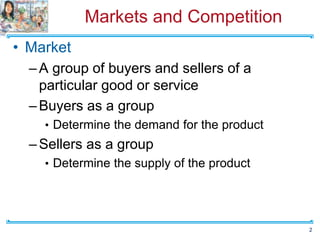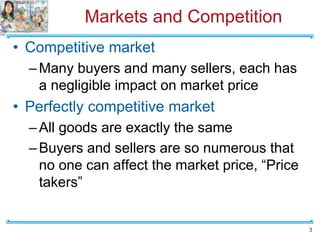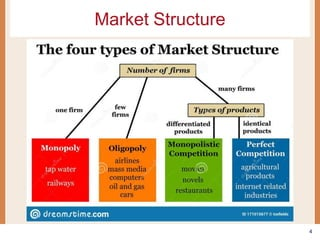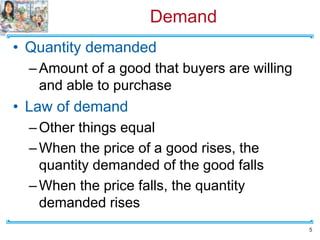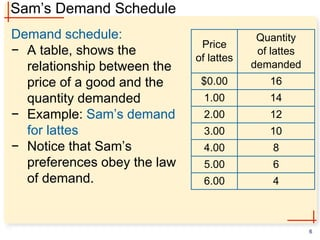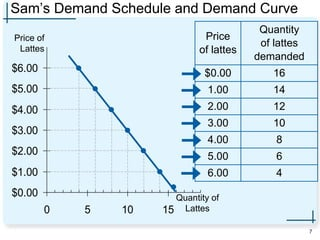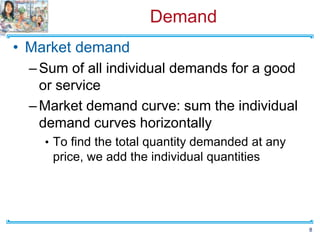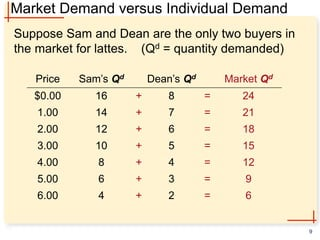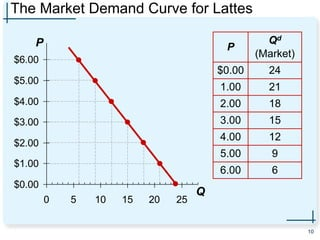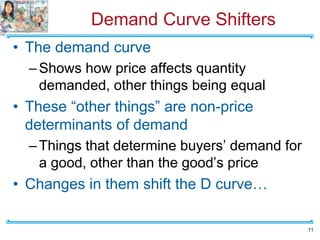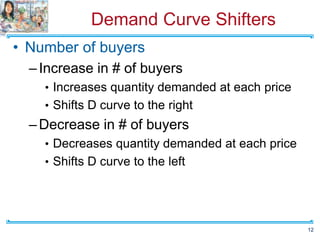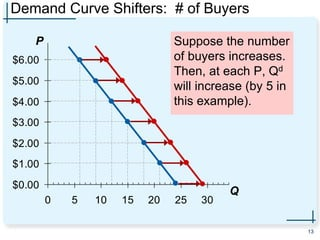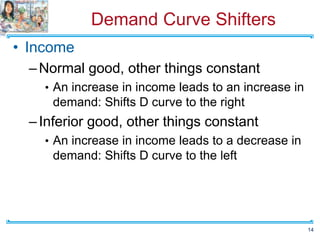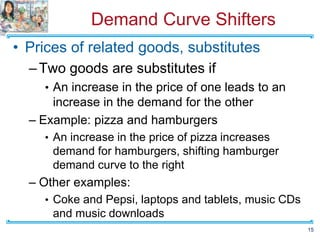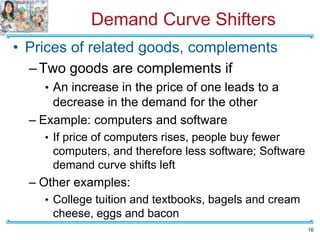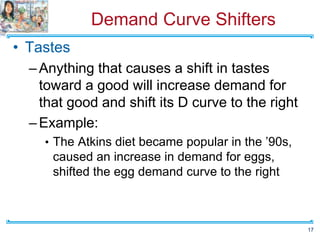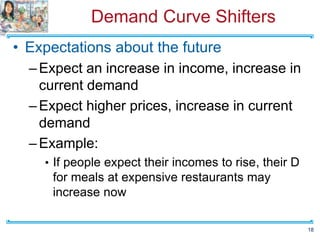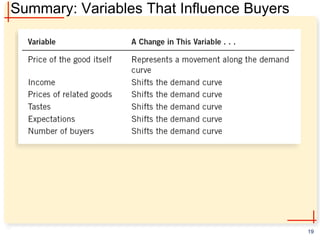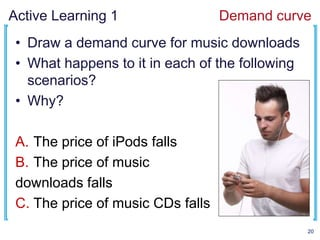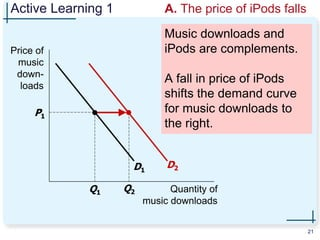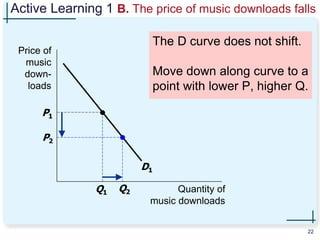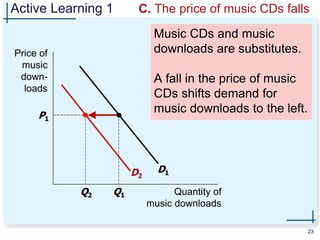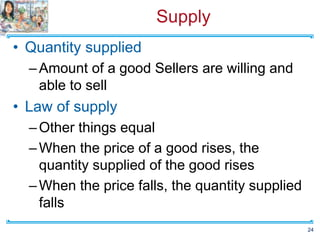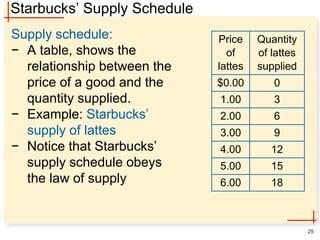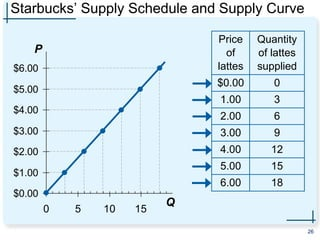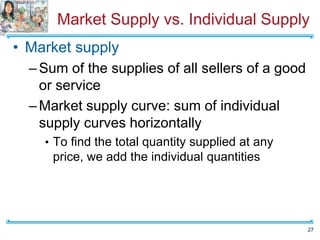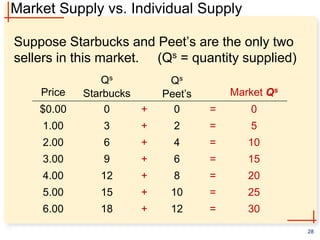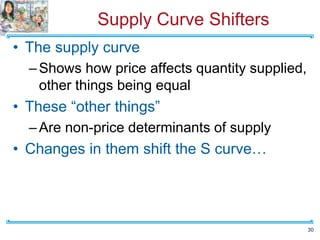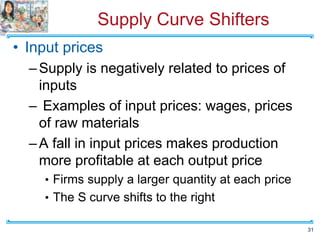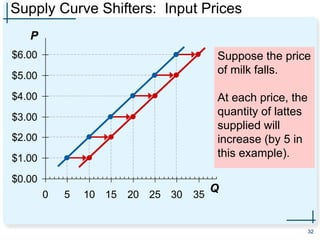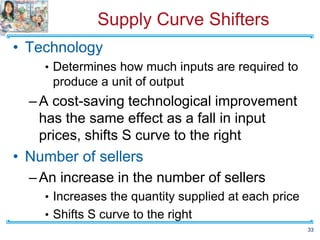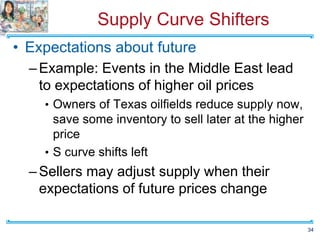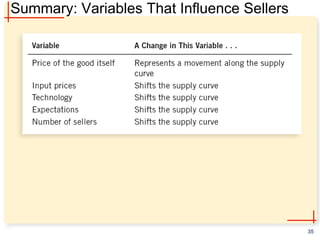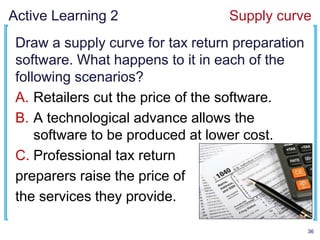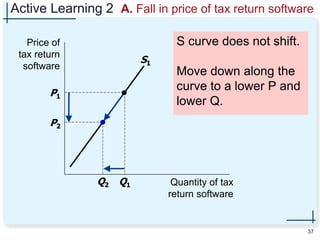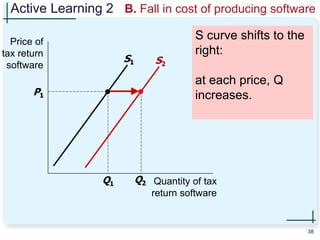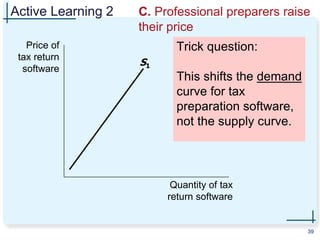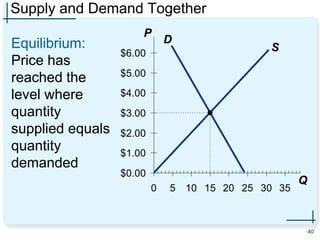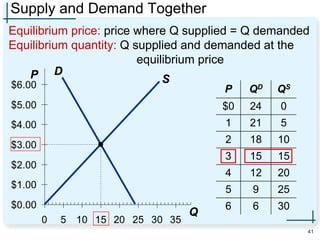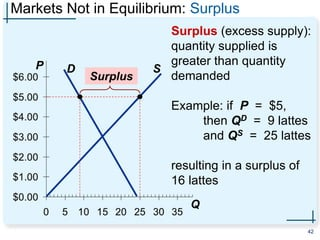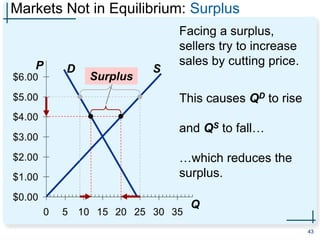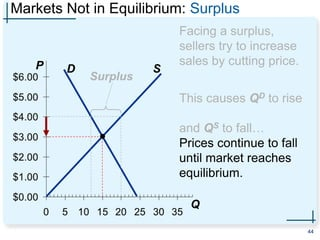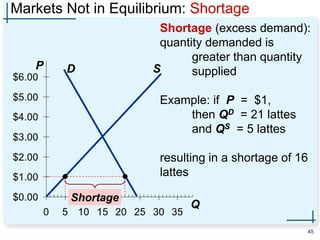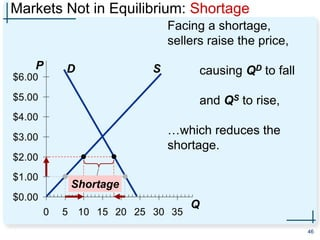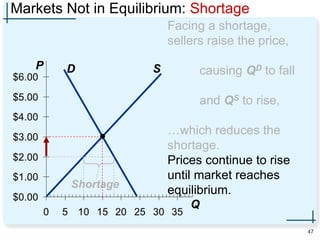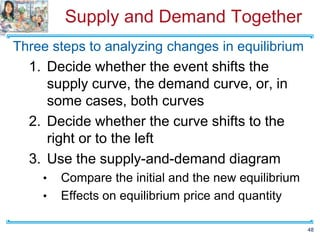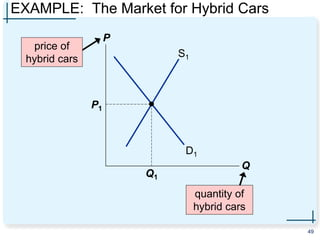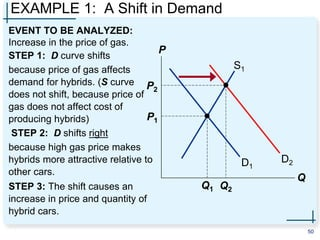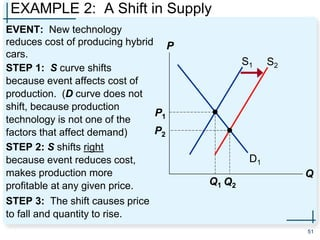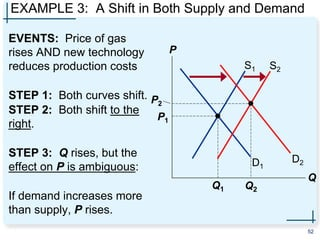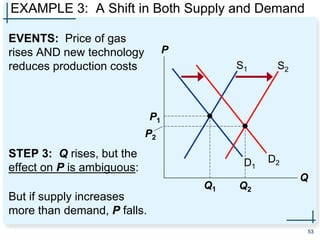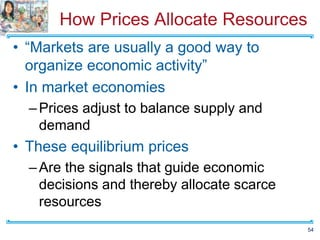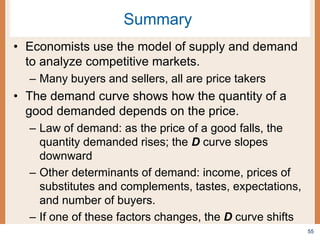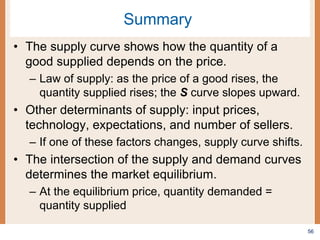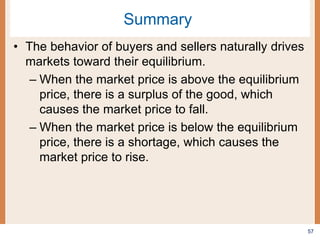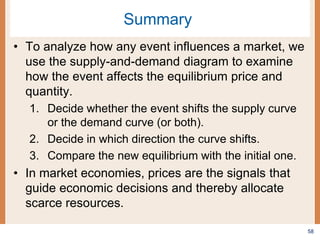1 sur 58
Publicité

### Chapter 4. (1).pptx

1. 1 The Market Forces of Supply and Demand The Principles of Economics Chapter 4 Jeyhun Isgandarli
2. Markets and Competition • Market –A group of buyers and sellers of a particular good or service –Buyers as a group • Determine the demand for the product –Sellers as a group • Determine the supply of the product 2
3. Markets and Competition • Competitive market –Many buyers and many sellers, each has a negligible impact on market price • Perfectly competitive market –All goods are exactly the same –Buyers and sellers are so numerous that no one can affect the market price, “Price takers” 3
4. Market Structure 4
5. Demand • Quantity demanded –Amount of a good that buyers are willing and able to purchase • Law of demand –Other things equal –When the price of a good rises, the quantity demanded of the good falls –When the price falls, the quantity demanded rises 5
6. Sam’s Demand Schedule Demand schedule: − A table, shows the relationship between the price of a good and the quantity demanded − Example: Sam’s demand for lattes − Notice that Sam’s preferences obey the law of demand. 6 Price of lattes Quantity of lattes demanded $0.00 16 1.00 14 2.00 12 3.00 10 4.00 8 5.00 6 6.00 4 7. Sam’s Demand Schedule and Demand Curve 7 Price of lattes Quantity of lattes demanded$0.00 16 1.00 14 2.00 12 3.00 10 4.00 8 5.00 6 6.00 4 $0.00$1.00 $2.00$3.00 $4.00$5.00 $6.00 0 5 10 15 Price of Lattes Quantity of Lattes 8. Demand • Market demand –Sum of all individual demands for a good or service –Market demand curve: sum the individual demand curves horizontally • To find the total quantity demanded at any price, we add the individual quantities 8 9. Market Demand versus Individual Demand Suppose Sam and Dean are the only two buyers in the market for lattes. (Qd = quantity demanded) 9 4 6 8 10 12 14 16 Sam’s Qd 2 3 4 5 6 7 8 Dean’s Qd + + + + = = = = 6 9 12 15 + = 18 + = 21 + = 24 Market Qd$0.00 6.00 5.00 4.00 3.00 2.00 1.00 Price
10. The Market Demand Curve for Lattes 10 $0.00$1.00 $2.00$3.00 $4.00$5.00 $6.00 0 5 10 15 20 25 P Q P Qd (Market)$0.00 24 1.00 21 2.00 18 3.00 15 4.00 12 5.00 9 6.00 6
11. Demand Curve Shifters • The demand curve –Shows how price affects quantity demanded, other things being equal • These “other things” are non-price determinants of demand –Things that determine buyers’ demand for a good, other than the good’s price • Changes in them shift the D curve… 11
12. Demand Curve Shifters • Number of buyers –Increase in # of buyers • Increases quantity demanded at each price • Shifts D curve to the right –Decrease in # of buyers • Decreases quantity demanded at each price • Shifts D curve to the left 12
13. Demand Curve Shifters: # of Buyers Suppose the number of buyers increases. Then, at each P, Qd will increase (by 5 in this example). 13 $0.00$1.00 $2.00$3.00 $4.00$5.00 $6.00 0 5 10 15 20 25 30 P Q 14. Demand Curve Shifters • Income –Normal good, other things constant • An increase in income leads to an increase in demand: Shifts D curve to the right –Inferior good, other things constant • An increase in income leads to a decrease in demand: Shifts D curve to the left 14 15. Demand Curve Shifters • Prices of related goods, substitutes –Two goods are substitutes if • An increase in the price of one leads to an increase in the demand for the other – Example: pizza and hamburgers • An increase in the price of pizza increases demand for hamburgers, shifting hamburger demand curve to the right – Other examples: • Coke and Pepsi, laptops and tablets, music CDs and music downloads 15 16. Demand Curve Shifters • Prices of related goods, complements –Two goods are complements if • An increase in the price of one leads to a decrease in the demand for the other – Example: computers and software • If price of computers rises, people buy fewer computers, and therefore less software; Software demand curve shifts left – Other examples: • College tuition and textbooks, bagels and cream cheese, eggs and bacon 16 17. Demand Curve Shifters • Tastes –Anything that causes a shift in tastes toward a good will increase demand for that good and shift its D curve to the right –Example: • The Atkins diet became popular in the ’90s, caused an increase in demand for eggs, shifted the egg demand curve to the right 17 18. Demand Curve Shifters • Expectations about the future –Expect an increase in income, increase in current demand –Expect higher prices, increase in current demand –Example: • If people expect their incomes to rise, their D for meals at expensive restaurants may increase now 18 19. Summary: Variables That Influence Buyers 19 20. Active Learning 1 Demand curve • Draw a demand curve for music downloads • What happens to it in each of the following scenarios? • Why? A. The price of iPods falls B. The price of music downloads falls C. The price of music CDs falls 20 21. Active Learning 1 A. The price of iPods falls Music downloads and iPods are complements. A fall in price of iPods shifts the demand curve for music downloads to the right. 21 Q2 Price of music down- loads Quantity of music downloads D1 D2 P1 Q1 22. Active Learning 1 B. The price of music downloads falls The D curve does not shift. Move down along curve to a point with lower P, higher Q. 22 Price of music down- loads Quantity of music downloads D1 P1 Q1 Q2 P2 23. Active Learning 1 C. The price of music CDs falls Music CDs and music downloads are substitutes. A fall in the price of music CDs shifts demand for music downloads to the left. 23 P1 Q1 Price of music down- loads Quantity of music downloads D1 D2 Q2 24. Supply • Quantity supplied –Amount of a good Sellers are willing and able to sell • Law of supply –Other things equal –When the price of a good rises, the quantity supplied of the good rises –When the price falls, the quantity supplied falls 24 25. Starbucks’ Supply Schedule Supply schedule: − A table, shows the relationship between the price of a good and the quantity supplied. − Example: Starbucks’ supply of lattes − Notice that Starbucks’ supply schedule obeys the law of supply 25 Price of lattes Quantity of lattes supplied$0.00 0 1.00 3 2.00 6 3.00 9 4.00 12 5.00 15 6.00 18
26. Starbucks’ Supply Schedule and Supply Curve 26 $0.00$1.00 $2.00$3.00 $4.00$5.00 $6.00 0 5 10 15 Price of lattes Quantity of lattes supplied$0.00 0 1.00 3 2.00 6 3.00 9 4.00 12 5.00 15 6.00 18 P Q
27. Market Supply vs. Individual Supply • Market supply –Sum of the supplies of all sellers of a good or service –Market supply curve: sum of individual supply curves horizontally • To find the total quantity supplied at any price, we add the individual quantities 27
28. Market Supply vs. Individual Supply Suppose Starbucks and Peet’s are the only two sellers in this market. (Qs = quantity supplied) 28 18 15 12 9 6 3 0 Qs Starbucks 12 10 8 6 4 2 0 Qs Peet’s + + + + = = = = 30 25 20 15 + = 10 + = 5 + = 0 Market Qs $0.00 6.00 5.00 4.00 3.00 2.00 1.00 Price 29. The Market Supply Curve 29$0.00 $1.00$2.00 $3.00$4.00 $5.00$6.00 0 5 10 15 20 25 30 35 P Q P QS (Market) $0.00 0 1.00 5 2.00 10 3.00 15 4.00 20 5.00 25 6.00 30 30. Supply Curve Shifters • The supply curve –Shows how price affects quantity supplied, other things being equal • These “other things” –Are non-price determinants of supply • Changes in them shift the S curve… 30 31. Supply Curve Shifters • Input prices –Supply is negatively related to prices of inputs – Examples of input prices: wages, prices of raw materials –A fall in input prices makes production more profitable at each output price • Firms supply a larger quantity at each price • The S curve shifts to the right 31 32. Supply Curve Shifters: Input Prices Suppose the price of milk falls. At each price, the quantity of lattes supplied will increase (by 5 in this example). 32$0.00 $1.00$2.00 $3.00$4.00 $5.00$6.00 0 5 10 15 20 25 30 35 P Q
33. Supply Curve Shifters • Technology • Determines how much inputs are required to produce a unit of output –A cost-saving technological improvement has the same effect as a fall in input prices, shifts S curve to the right • Number of sellers –An increase in the number of sellers • Increases the quantity supplied at each price • Shifts S curve to the right 33
34. Supply Curve Shifters • Expectations about future –Example: Events in the Middle East lead to expectations of higher oil prices • Owners of Texas oilfields reduce supply now, save some inventory to sell later at the higher price • S curve shifts left –Sellers may adjust supply when their expectations of future prices change 34
35. Summary: Variables That Influence Sellers 35
36. Active Learning 2 Supply curve Draw a supply curve for tax return preparation software. What happens to it in each of the following scenarios? A. Retailers cut the price of the software. B. A technological advance allows the software to be produced at lower cost. C. Professional tax return preparers raise the price of the services they provide. 36
37. Active Learning 2 A. Fall in price of tax return software S curve does not shift. Move down along the curve to a lower P and lower Q. 37 Price of tax return software Quantity of tax return software S1 P1 Q1 Q2 P2
38. Active Learning 2 B. Fall in cost of producing software S curve shifts to the right: at each price, Q increases. 38 Price of tax return software Quantity of tax return software S1 P1 Q1 S2 Q2
39. Active Learning 2 C. Professional preparers raise their price Trick question: This shifts the demand curve for tax preparation software, not the supply curve. 39 Price of tax return software Quantity of tax return software S1
40. Supply and Demand Together Equilibrium: Price has reached the level where quantity supplied equals quantity demanded 40 $0.00$1.00 $2.00$3.00 $4.00$5.00 $6.00 0 5 10 15 20 25 30 35 P Q D S 41. Supply and Demand Together Equilibrium price: price where Q supplied = Q demanded Equilibrium quantity: Q supplied and demanded at the equilibrium price 41 P QD QS$0 24 0 1 21 5 2 18 10 3 15 15 4 12 20 5 9 25 6 6 30 $0.00$1.00 $2.00$3.00 $4.00$5.00 $6.00 0 5 10 15 20 25 30 35 P Q D S 42. Markets Not in Equilibrium: Surplus Surplus (excess supply): quantity supplied is greater than quantity demanded Example: if P =$5, then QD = 9 lattes and QS = 25 lattes resulting in a surplus of 16 lattes 42 $0.00$1.00 $2.00$3.00 $4.00$5.00 $6.00 0 5 10 15 20 25 30 35 P Q D S Surplus 43. Markets Not in Equilibrium: Surplus Facing a surplus, sellers try to increase sales by cutting price. This causes QD to rise and QS to fall… …which reduces the surplus. 43$0.00 $1.00$2.00 $3.00$4.00 $5.00$6.00 0 5 10 15 20 25 30 35 P Q D S Surplus
44. Markets Not in Equilibrium: Surplus Facing a surplus, sellers try to increase sales by cutting price. This causes QD to rise and QS to fall… Prices continue to fall until market reaches equilibrium. 44 $0.00$1.00 $2.00$3.00 $4.00$5.00 $6.00 0 5 10 15 20 25 30 35 P Q D S Surplus 45. Markets Not in Equilibrium: Shortage Shortage (excess demand): quantity demanded is greater than quantity supplied Example: if P =$1, then QD = 21 lattes and QS = 5 lattes resulting in a shortage of 16 lattes 45 $0.00$1.00 $2.00$3.00 $4.00$5.00 $6.00 0 5 10 15 20 25 30 35 P Q D S Shortage 46. Markets Not in Equilibrium: Shortage Facing a shortage, sellers raise the price, causing QD to fall and QS to rise, …which reduces the shortage. 46$0.00 $1.00$2.00 $3.00$4.00 $5.00$6.00 0 5 10 15 20 25 30 35 P Q D S Shortage
47. Markets Not in Equilibrium: Shortage Facing a shortage, sellers raise the price, causing QD to fall and QS to rise, …which reduces the shortage. Prices continue to rise until market reaches equilibrium. 47 $0.00$1.00 $2.00$3.00 $4.00$5.00 $6.00 0 5 10 15 20 25 30 35 P Q D S Shortage 48. Supply and Demand Together Three steps to analyzing changes in equilibrium 1. Decide whether the event shifts the supply curve, the demand curve, or, in some cases, both curves 2. Decide whether the curve shifts to the right or to the left 3. Use the supply-and-demand diagram • Compare the initial and the new equilibrium • Effects on equilibrium price and quantity 48 49. EXAMPLE: The Market for Hybrid Cars 49 P Q D1 S1 P1 Q1 price of hybrid cars quantity of hybrid cars 50. EXAMPLE 1: A Shift in Demand EVENT TO BE ANALYZED: Increase in the price of gas. STEP 1: D curve shifts because price of gas affects demand for hybrids. (S curve does not shift, because price of gas does not affect cost of producing hybrids) STEP 2: D shifts right because high gas price makes hybrids more attractive relative to other cars. STEP 3: The shift causes an increase in price and quantity of hybrid cars. 50 P Q D1 S1 P1 Q1 D2 P2 Q2 51. EXAMPLE 2: A Shift in Supply EVENT: New technology reduces cost of producing hybrid cars. STEP 1: S curve shifts because event affects cost of production. (D curve does not shift, because production technology is not one of the factors that affect demand) STEP 2: S shifts right because event reduces cost, makes production more profitable at any given price. STEP 3: The shift causes price to fall and quantity to rise. 51 P Q D1 S1 P1 Q1 S2 P2 Q2 52. EXAMPLE 3: A Shift in Both Supply and Demand EVENTS: Price of gas rises AND new technology reduces production costs STEP 1: Both curves shift. STEP 2: Both shift to the right. STEP 3: Q rises, but the effect on P is ambiguous: If demand increases more than supply, P rises. 52 P Q D1 S1 P1 Q1 S2 D2 P2 Q2 53. EXAMPLE 3: A Shift in Both Supply and Demand EVENTS: Price of gas rises AND new technology reduces production costs STEP 3: Q rises, but the effect on P is ambiguous: But if supply increases more than demand, P falls. 53 P Q D1 S1 P1 Q1 S2 D2 P2 Q2 54. How Prices Allocate Resources • “Markets are usually a good way to organize economic activity” • In market economies –Prices adjust to balance supply and demand • These equilibrium prices –Are the signals that guide economic decisions and thereby allocate scarce resources 54 55. Summary • Economists use the model of supply and demand to analyze competitive markets. – Many buyers and sellers, all are price takers • The demand curve shows how the quantity of a good demanded depends on the price. – Law of demand: as the price of a good falls, the quantity demanded rises; the D curve slopes downward – Other determinants of demand: income, prices of substitutes and complements, tastes, expectations, and number of buyers. – If one of these factors changes, the D curve shifts 55 56. Summary • The supply curve shows how the quantity of a good supplied depends on the price. – Law of supply: as the price of a good rises, the quantity supplied rises; the S curve slopes upward. • Other determinants of supply: input prices, technology, expectations, and number of sellers. – If one of these factors changes, supply curve shifts. • The intersection of the supply and demand curves determines the market equilibrium. – At the equilibrium price, quantity demanded = quantity supplied 56 57. Summary • The behavior of buyers and sellers naturally drives markets toward their equilibrium. – When the market price is above the equilibrium price, there is a surplus of the good, which causes the market price to fall. – When the market price is below the equilibrium price, there is a shortage, which causes the market price to rise. 57 58. Summary • To analyze how any event influences a market, we use the supply-and-demand diagram to examine how the event affects the equilibrium price and quantity. 1. Decide whether the event shifts the supply curve or the demand curve (or both). 2. Decide in which direction the curve shifts. 3. Compare the new equilibrium with the initial one. • In market economies, prices are the signals that guide economic decisions and thereby allocate scarce resources. 58 ### Notes de l'éditeur 1. Notes about Chapter 1: Most instructors try to cover this chapter in a single class session (especially those that are teaching the second of a two-semester sequence). If you are teaching principles of microeconomics, you might consider skipping Principles 8-10, which deal with macroeconomics. 2. In the real world, there are relatively few perfectly competitive markets. Most goods come in lots of different varieties—including ice cream, the example in the textbook. And there are many markets in which the number of firms is small enough that some of them have the ability to affect the market price. For now, though, we look at supply and demand in perfectly competitive markets, for two reasons: First, it’s easier to learn. Understanding perfectly competitive markets makes it a lot easier to learn the more realistic but complicated analysis of imperfectly competitive markets. Second, despite the lack of realism, the perfectly competitive model can teach us a lot about how the world works, as we will see many times in the chapters that follow. 3. Demand shows the relationship between the price of a good and quantity demanded. It can be represented with: - A demand schedule: a table - A demand curve: a graph (with price on the vertical axis and quantity on the horizontal axis) Helen’s demand is the individual demand (an individual’s demand for a product) 4. This example violates the “many buyers” condition of perfect competition. Yet, we are merely trying to show here that, at each price, the quantity demanded in the market is the sum of the quantity demanded by each buyer in the market. This holds whether there are two buyers or two million buyers. But it would be harder to fit data for two million buyers on this slide, so we settle for two. 5. Income is the first demand shifter discussed in this chapter of the textbook. I chose to start with a different one (number of buyers), for the following reason: In discussing the impact of changes in income on the demand curve, the textbook also introduces the concept of normal goods and inferior goods. Students may find it easier to learn about curve shifts if the presentation focuses solely on a curve shift (at least initially) without simultaneously introducing other concepts. If you wish to present the demand shifters in the same order as they appear in the book, simply reorder the slides in this presentation. 6. Beginning economics students often have trouble understanding the difference between a movement along the curve and a shift in the curve. Here, the animation has been carefully designed to help students see that a shift in the curve results from an increase in quantity at each price. (A more realistic scenario would involve a non-parallel shift, where the horizontal distance of the shift would be greater for lower prices than higher ones. However, to remain consistent with the textbook, and to keep things simple, this slide shows a parallel shift.) 7. If you are willing to spend a couple extra minutes on substitutes and complements, and have a blackboard or whiteboard to draw on, here’s an idea: Before (or instead of) showing this slide, draw the demand curve for hamburgers. Pick a price, say$5, and draw a horizontal line at that price, extending from the vertical axis through the D curve and continuing to the right. Suppose Q = 1000 when P = \$5. Label this on the horizontal axis. Now ask your students: If pizza becomes more expensive, but price of hamburgers does not change, what would happen to the quantity of hamburgers demanded? Would it remain at 1000, would it increase, or would it decrease? Explain. Some and perhaps most students will see right away that people will want more hamburgers when the price of pizza rises. After establishing this, note that the increase in the price of pizza caused an increase in the quantity demanded of hamburgers. Then state the term “substitutes” and give the definition. Before giving the other examples (listed in the last bullet of this slide), do a similar exercise to develop the concept of complements. Finally, give the examples of substitutes and complements from the last bullet point of this and the following slide, but mix up the order and ask students to identify whether each example is complements or substitutes.
8. Additional example: If the economy sours and people worry about their future job security, D for new cars may fall now
9. Students should notice that the only determinant of quantity demanded that causes a movement along the curve is price. Also notice: price is one of the variables measured along the axes of the graph. Here’s a handy rule of thumb students can use to remember whether the curve shifts: If the variable causing demand to change is measured on one of the axes, you move along the curve. If the variable that’s causing demand to change does not appear on either axis, then the curve shifts. This rule of thumb works with all curves in economics that involve an X-Y relationship, including the supply curve, the marginal cost curve, the IS and LM curves (not covered in this book), and many others, though it does not apply to curves drawn on time series graphs. For an interesting break, you can discuss the case study ‘Two ways to reduce the quantity of smoking demanded’ on how to reduce smoking: 1. Use public service announcements, mandatory health warnings on cigarette packages, and prohibition of cigarette advertising on television to decrease the demand for cigarettes and other tobacco products. 2. Tax the manufacturer, and decreasing the quantity demanded (movement along demand curve). Studies showed: 10% ↑ in price → 4% ↓ in smoking Teenagers: 10% ↑ in price → 12% ↓ in smoking
10. In each case, there are only three possible answers: - The curve shifts to the right - The curve shifts to the left - The curve does not shift (though there may be a movement along the curve)
11. Point out to your students that there are no numbers or units on either axis, and we are using “P1” and “Q1” to represent the initial price and quantity, rather than specific numerical values. Tell them that this is common, because in much economic analysis, the goal is only to see the direction of changes, not specific amounts. (Besides, if we put numbers on this graph, they’d just have been made up, so why bother?) Also point out the following: The price of music downloads is the same, but the quantity demanded is now higher. In fact, this is the nature of a shift in a curve: at any given price, the quantity is different than before.
12. Supply comes from the behavior of sellers.
13. Supply shows the relationship between the price of a good and quantity supplied. It can be represented with: - A supply schedule: a table - A supply curve: a graph (with price on the vertical axis and quantity on the horizontal axis) Starbucks’ supply is the individual supply(an individual producer’s supply for a product)
14. Again, the assumption of only two sellers is a clear violation of perfect competition. However, it’s much easier for students to learn how the market supply curve relates to individual supplies in the two-seller case.
15. “Non-price determinants of supply” simply means the things—other than the price of a good—that determine sellers’ supply of the good.
16. “Output price” just means the price of the good that firms are producing and selling. I have used “output price” here to distinguish it from “input prices.”
17. Again, the animation here is carefully designed to help make clear that a shift in the supply curve means that there is a change in the quantity supplied at each possible price. If it seems tedious, you can turn it off. In any case, be assured that, by the end of this chapter, the animation of curve shifts will be streamlined and simplified.
18. “Tax return preparation software” means programs like TurboTax by Quicken and TaxCut by H&R Block.
19. Professional tax prepares are a substitute for a tax preparation software (we use at home). A change in the price of a good will shift the demand for the substitute.
20. In equilibrium, various forces are in balance. A situation in which market price has reached the level where Quantity supplied = Quantity demanded; where supply and demand curves intersect
21. Step 1 requires knowing all of the things that can shift D and S—the non-price determinants of demand and of supply.
22. Notice: When P rises, producers supply a larger quantity of hybrids, even though the S curve has not shifted. Always be careful to distinguish b/w a shift in a curve and a movement along the curve.
23. In the textbook, the conclusion of this chapter offers some very nice elaboration on the third bullet point.
Publicité